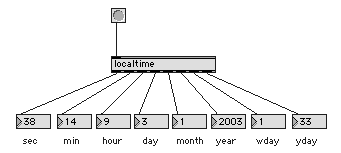# Digital Music Programming II: localtime

This lab demonstrates how to get the current time from the computer and how to generate multiple integer outputs to an object. Here is a picture of the final object and an example usage:The outputs from the localtime object are as follows:

1. second (0-59)
2. minute (0-59)
3. hour (0-23)
4. day (1-31)
5. month (1-12) -- Jan = 1, etc.
6. year -- e.g.: 2003.
7. wday (0-6) -- day of the week with Sunday being day 0.
8. yday (0-365/6) -- day of the year with Jan 1st being day 0.

You may either bang the input or provied an integer which is the Epoch time in seconds since midnight, Jan. 1, 1970. If a bang is used, then the current time from the operating system will be calculated.

The order in which outputs are declared control the order of the outputs on a Max object. The first object declared is the right-most outlet. The last object declared is the left-most object. For example, the following code:

```   mo->outputYday  = intout(mo);
mo->outputWday  = intout(mo);
mo->outputYear  = intout(mo);
mo->outputMonth = intout(mo);
mo->outputDay   = intout(mo);
mo->outputHour  = intout(mo);
mo->outputMin   = intout(mo);
mo->outputSec   = intout(mo);
```
will generate the following ports, numbered from left to right on the bottom of the Max object:
1. sec
2. min
3. hour
4. day
5. month
6. year
7. wday
8. yday

localtime.c
 ``` #include "ext.h" #include typedef struct { t_object maxData; void* outputSec; void* outputMin; void* outputHour; void* outputDay; void* outputMonth; void* outputYear; void* outputWday; void* outputYday; } MyObject; void* object_data = NULL; void* create_object (void); void InputEpochTime (MyObject* mo, long timeinsec); void InputBang (MyObject* mo); void OutputData (MyObject* mo, struct tm* timestruct); void main(void) { setup((t_messlist**)&object_data, (method)create_object, NULL, sizeof(MyObject), NULL, A_NOTHING); addint ((method)InputEpochTime); addbang((method)InputBang); } void* create_object(void) { MyObject *mo; mo = (MyObject*)newobject(object_data); mo->outputYday = intout(mo); mo->outputWday = intout(mo); mo->outputYear = intout(mo); mo->outputMonth = intout(mo); mo->outputDay = intout(mo); mo->outputHour = intout(mo); mo->outputMin = intout(mo); mo->outputSec = intout(mo); return mo; } void InputEpochTime(MyObject* mo, long timeinsec) { time_t currenttime = timeinsec; struct tm* timestruct = localtime(¤ttime); OutputData(mo, timestruct); } void InputBang(MyObject* mo) { time_t currenttime = time(NULL); struct tm* timestruct = localtime(¤ttime); OutputData(mo, timestruct); } void OutputData(MyObject* mo, struct tm* timestruct) { outlet_int(mo->outputSec, timestruct->tm_sec); outlet_int(mo->outputMin, timestruct->tm_min); outlet_int(mo->outputHour, timestruct->tm_hour); outlet_int(mo->outputDay, timestruct->tm_mday); outlet_int(mo->outputMonth, timestruct->tm_mon); outlet_int(mo->outputYear, timestruct->tm_year + 1900); outlet_int(mo->outputWday, timestruct->tm_wday); outlet_int(mo->outputYday, timestruct->tm_yday); } ```